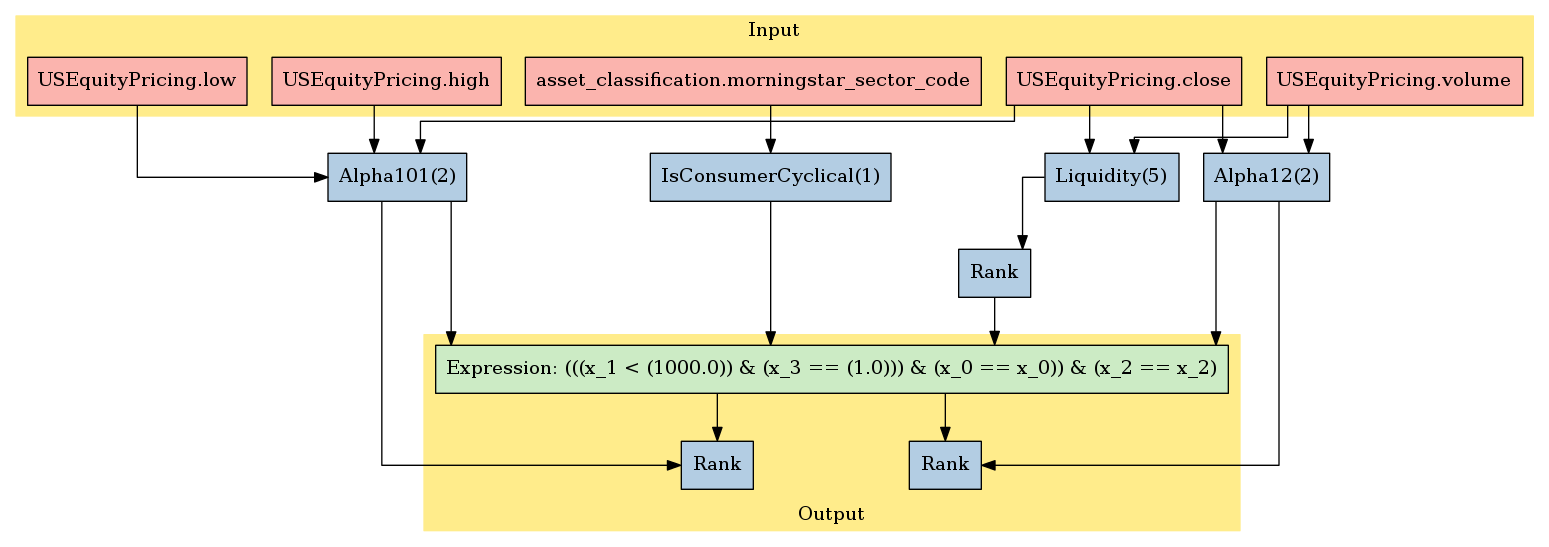Notebook

Alpha Template¶

In :
from quantopian.pipeline import Pipeline
from quantopian.pipeline import CustomFactor
from quantopian.research import run_pipeline
from quantopian.pipeline.data import morningstar
from quantopian.pipeline.data.builtin import USEquityPricing
from quantopian.pipeline.factors import Latest
import numpy as np
In :
class Alpha101(CustomFactor):
inputs = [USEquityPricing.close, USEquityPricing.high, USEquityPricing.low]
window_length = 2

def compute(self, today, assets, out, close, high, low):

# Using close of t-1 as the t open. Workaround for known open bug.
out[:] = (close[-1] - close[-2]) / ((high[-1] - low[-1]) + .001)
In :
class Alpha12(CustomFactor):
#(sign(delta(volume, 1)) * (-1 * delta(close, 1)))

inputs = [USEquityPricing.volume, USEquityPricing.close]
window_length = 2

def compute(self, today, assets, out, volume, close):
out[:] = np.sign(volume[-1] - volume[-2]) * -1 * (close[-1] - close[-2])
In :
class Liquidity(CustomFactor):
inputs = [USEquityPricing.volume, USEquityPricing.close]
window_length = 5

def compute(self, today, assets, out, volume, close):
out[:] = (volume * close).mean(axis=0)
In :
class IsConsumerCyclical(CustomFactor):
inputs = [morningstar.asset_classification.morningstar_sector_code]
window_length = 1
def compute(self, today, assets, out, adr):
out[:] = adr[-1] == 102
In :
alpha101 = Alpha101()
alpha12 = Alpha12()
liquidity = Liquidity()
is_cc = IsConsumerCyclical()

liquidity_rank = liquidity.rank(ascending=False)
ok_universe = (1000 > liquidity_rank) & is_cc.eq(1) & alpha101.eq(alpha101) & alpha12.eq(alpha12)

alpha101_rank = alpha101.rank(mask=ok_universe,  ascending=False)
alpha12_rank = alpha12.rank(mask=ok_universe,  ascending=False)
In :
pipe = Pipeline()

pipe.set_screen(ok_universe)
In :
pipe.show_graph(format='png')
Out:In :
daily_alpha_ranks = run_pipeline(pipe, start_date='2015-11-20', end_date='2015-11-25')
In :